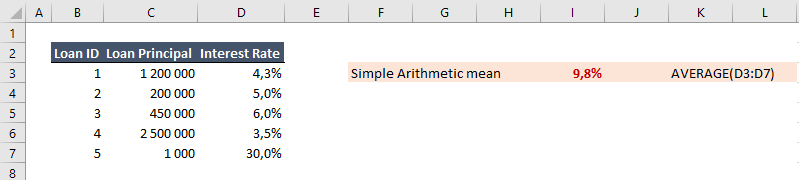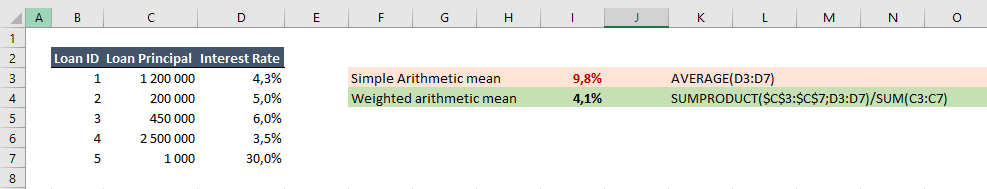• 2. 2. 2020
• Ing. Jan Zedníček - Data & Finance
• 0

Weighted arithmetic mean is a statistical value of broad use not only in mathematics or statistics. If you need to calculate weighted arithmetic mean in Excel, here is a guide

## How Works Function SUMPRODUCT and Weighted Arithmetic Mean in Excel

Example: Lets have few loans where every loan has different principal and interest rate. The task is to calculate average interest rate of loans.Basic arithmetic mean gives us 9,8% which does not make sense since all loans have different weight (exploitation). Basic arithmetic mean influences last loan only up to 1 000 with high interest rate.

Solution is to use weighted arithmetic mean where the weights is Principal. Function SUMPRODUCT is used to do just that. It will give us the correct rate for the whole loan portfolio.Rate this post
Tags:### Ing. Jan Zedníček - Data & Finance

My name is Jan Zedníček and I work as a freelancer. I have been working as a freelancer for many companies for more than 10 years.
I used to work as a financial controller, analyst and manager at many different companies in field of banking and manufacturing. When I am not at work, I like playing volleyball, chess, doing a workout in the gym.